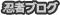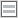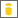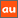# 0除算に関するアナウンスメントを公開しています

The purpose of this site is to publish announcements on the theory of division by zero

## Announcement 410: What is mathematics? -- beyond logic; for great challengers on the division by zero

Institute of Reproducing Kernels
Kawauchi-cho, 5-1648-16,
Kiryu 376-0041, Japan
January 24, 2018

The Institute of Reproducing Kernels is dealing with the theory of divisionby zero calculus and declares that the division by zero was discovered as $0/0 =1/0 = z/0 = 0$ in a natural sense on 2014.2.2. The result shows a new basic idea on the universe and space since Aristotels $($BC384 - BC322$)$ and Euclid $($BC 3 Century - $)$, and the division by zero is since Brahmagupta $($598 - 668?$)$. In particular, Brahmagupta defined as $0/0 = 0$ in Brhmasphuasiddhnta $($628$)$, however, our world history stated that his definition $0/0 = 0$ is wrong over 1300 years, but, we showed that his definition is suitable. For the details, see the references and the site: http://okmr.yamatoblog.net/
We wrote a global book manuscript  with 154 pages and stated that the division by zero is trivial and clear, and in the last section of the manuscript we stated as follows:

Conclusion
Apparently, the common sense on the division by zero with a long and mysterious history is wrong and our basic idea on the space around the point at infinity is also wrong since Euclid. On the gradient or on derivatives we have a great missing since $\tan(π/2) = 0$. Our mathematics is also wrong in elementary mathematics on the division by zero.
This book is an elementary mathematics on our division by zero as the first publication of books for the topics. The contents have wide connections to various fields beyond mathematics. The author expects the readers write some philosophy, papers and essays on the division by zero from this simple source book.
The division by zero theory may be developed and expanded greatly as in the author’s conjecture whose break theory was recently given surprisingly and deeply by Professor Qi’an Guan  since 30 years proposed in  $($the original is in $)$.
We have to arrange globally our modern mathematics with our division by zero in our undergraduate level.
We have to change our basic ideas for our space and world.
We have to change globally our textbooks and scientific books on the division by zero.

However, we have still curious situations and opinions for us on the division by zero; in particular, the two great challengers Jakub Czajko and Ilija Baruki on the division by zero in connection with physics stated that we do not have the definition of the division $0/0$, however $0/0 = 1$. They seem to think that a truth is based on physical objects and is not on our mathematics. In such a cases, we will not be able to continue discussions on the division by zero more, because for mathematicians, they will not be able to follow their logics more. However, then we will ask for the question that what are the values and contributions of your articles and discussions. We
will expect some contributions, of course.
This question will reflect to mathematicians contrary. We stated for the estimation of mathematisc in : Mathematics is the collection of relations and, good results are fundamental, beautiful, and give great good impacts to human beings.
With this estimation, we stated that the Euler formula
$$e^{πi} = −1$$
is the best result in mathematics in details in:
No.81, May 2012$($pdf 432kb$)$ www.jams.or.jp/kaiho/kaiho-81.pdf
In order to show the importance of our division by zero and division by zero calculus we are requested to show their importance.
It seems that the long and mysterious confusions for the division by zero is on the definition. – Indeed, when we consider the division by zero $a/0$ in the usual sense of the fundamental equation 0 · $z = a$, we have immediately the simple contradiction, however, we have such cases may happen, in particular, in mathematical formulas and physical formulas on the universe.

References
 I. Baruki, Dialectical Logic Negation Of Classical Logic, http://vixra.org/abs/1801.0256
 J. Czajko, Algebraic division by zero implemented as quasigeometric multiplication by infinity in real and complex multispatial hyperspaces, Available online at www.worldscientificnews.com WSN 92$($2$)$ $($2018$)$ 171-197
 Q. Guan, A proof of Saitoh’s conjecture for conjugate Hardy H2 kernels, arXiv:1712.04207.
 M. Kuroda, H. Michiwaki, S. Saitoh, and M. Yamane, New meanings of the division by zero and interpretations on $100/0 = 0$ and on $0/0 = 0$, Int. J. Appl. Math. 27 $($2014$)$, no 2, pp. 191-198, DOI: 10.12732/ijam.v27i2.9.
 T. Matsuura and S. Saitoh, Matrices and division by zero  $z/0=0$, Advances in Linear Algebra & Matrix Theory, 6$($2016$)$, 51-58 Published Online June 2016 in SciRes. http://www.scirp.org/journal/alamt http://dx.doi.org/10.4236/alamt.2016.62007.
 T. Matsuura and S. Saitoh, Division by zero calculus and singular integrals. $($Submitted for publication$)$
 T. Matsuura, H. Michiwaki and S. Saitoh, $\log0 = \log\infty = 0$ and applications. Differential and Difference Equations with Applications. Springer Proceedings in Mathematics & Statistics.
 H. Michiwaki, S. Saitoh and M.Yamada, Reality of the division by zero z/0 = 0. IJAPM International J. of Applied Physics and Math. 6$($2015$)$, 1–8. http://www.ijapm.org/show-63-504-1.html
 H. Michiwaki, H. Okumura and S. Saitoh, Division by Zero $z/0 = 0$ in Euclidean Spaces, International Journal of Mathematics and Computation, 28$($2017$)$; Issue 1 1-16.
 H. Okumura, S. Saitoh and T. Matsuura, Relations of 0 and ∞, Journal of Technology and Social Science $($JTSS$)$, 1$($2017$)$, 70-77.
 H. Okumura and S. Saitoh, The Descartes circles theorem and division by zero calculus. https://arxiv.org/abs/1711.04961 (2017.11.14).
 H. Okumura, Wasan geometry with the division by 0. https://arxiv.org/abs/1711.06947 International Journal of Geometry.
 H. Okumura and S. Saitoh, Applications of the division by zero calculus to Wasan geometry. $($Submitted for publication$)$.
 S. Pinelas and S. Saitoh, Division by zero calculus and differential equations. Differential and Difference Equations with Applications. Springer Proceedings in Mathematics & Statistics.
 H. G. Romig, Discussions: Early History of Division by Zero, American Mathematical Monthly, Vol. 31, No. 8. $($Oct., 1924$)$, pp. 387-389.
 T. M. Rassias, Editor, Nonlinear Mathematical Analysis and Applications, HadronicPress,Palm Harbor,FL34682-1577,USA:ISBN1-57485-044-X,1998,  pp.223234: Nonlinear transforms and analyticity of functions, Saburou Saitoh.
 S. Saitoh, The Bergman norm and the Szegö norm, Trans. Amer. Math. Soc. 249 $($1979$)$, no. 2, 261–279.
 S. Saitoh, Theory of reproducing kernels and its applications. Pitman Research Notes in Mathematics Series, 189. Longman Scientific & Technical, Harlow; copublished in the United States with John Wiley & Sons, Inc., New York, 1988. x+157 pp. ISBN: 0-582-03564-3
 S. Saitoh, Generalized inversions of Hadamard and tensor products for matrices, Advances in Linear Algebra & Matrix Theory. 4 $($2014$)$, no. 2, 87–95. http://www.scirp.org/journal/ALAMT/
 S. Saitoh, A reproducing kernel theory with some general applications, Qian,T./Rodino,L.$($eds.$)$: Mathematical Analysis, Probability and Applications - Plenary Lectures: Isaac 2015, Macau, China, Springer Proceedings in Mathematics and Statistics, 177$($2016$)$, 151-182. $($Springer$)$
. S. Saitoh, Mysterious Properties of the Point at Infinity. arXiv:1712.09467 [math.GM]$($2017.12.17$)$.
 S. Saitoh, Division by zero calculus $($154 pages: draft$)$: $($http://okmr.yamatoblog.net/ $)$
 S.-E. Takahasi, M. Tsukada and Y. Kobayashi, Classification of continuous fractional binary operations on the real and complex fields, Tokyo Journal of Mathematics, 38(2015), no. 2, 369-380.

PR

### コメント

お名前 Black LimeGreen SeaGreen Teal FireBrick Tomato IndianRed BurlyWood SlateGray DarkSlateBlue LightPink DeepPink DarkOrange Gold DimGray Silverプロフィール

ＨＮ：